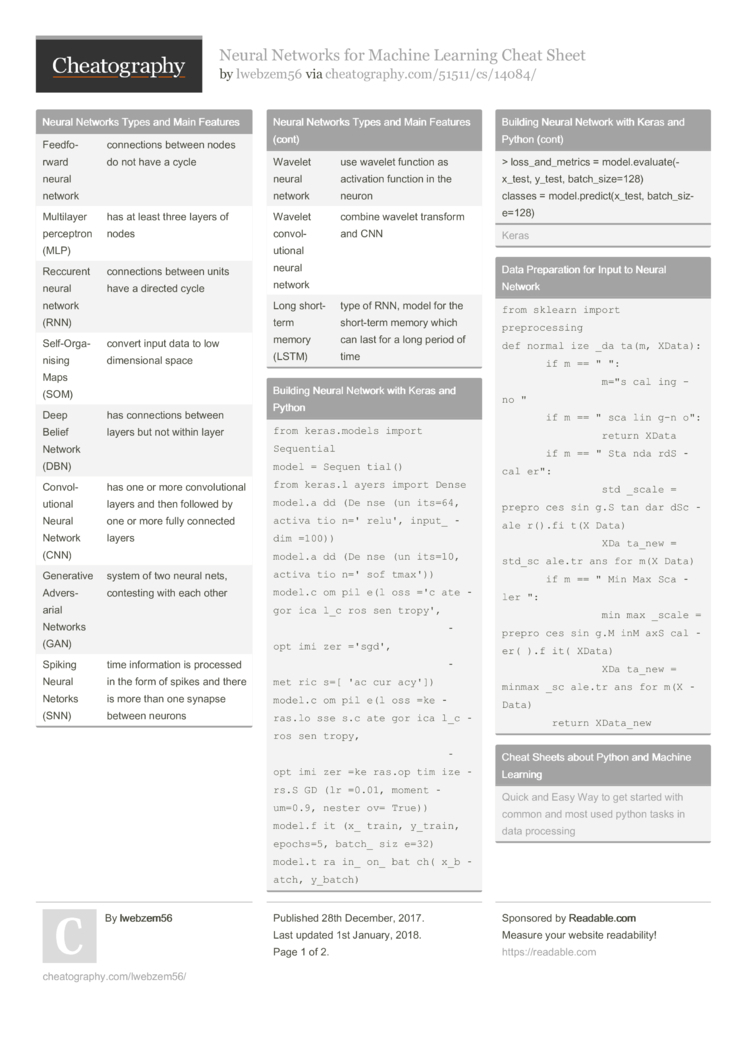# Neural Networks for Machine Learning Cheat Sheet by lwebzem56

Neural Networks for Machine Learning - showing neural networks types, applications, weight updates, python source code and links.

### Neural Networks Types and Main Features

 Feedfo­rward neural network connec­tions between nodes do not have a cycle Multilayer perceptron (MLP) has at least three layers of nodes Reccurent neural network (RNN) connec­tions between units have a directed cycle Self-O­rga­nising Maps (SOM) convert input data to low dimens­ional space Deep Belief Network (DBN) has connec­tions between layers but not within layer Convol­utional Neural Network (CNN) has one or more convol­utional layers and then followed by one or more fully connected layers Generative Advers­arial Networks (GAN) system of two neural nets, contesting with each other Spiking Neural Netorks (SNN) time inform­ation is processed in the form of spikes and there is more than one synapse between neurons Wavelet neural network use wavelet function as activation function in the neuron Wavelet convol­utional neural network combine wavelet transform and CNN Long short-term memory (LSTM) type of RNN, model for the short-term memory which can last for a long period of time

### Building Neural Network with Keras and Python

 ```from keras.models import Sequential model = Sequential() from keras.layers import Dense model.add(Dense(units=64, activation='relu', input_dim=100)) model.add(Dense(units=10, activation='softmax')) model.compile(loss='categorical_crossentropy',               optimizer='sgd',               metrics=['accuracy']) model.compile(loss=keras.losses.categorical_crossentropy,               optimizer=keras.optimizers.SGD(lr=0.01, momentum=0.9, nesterov=True)) model.fit(x_train, y_train, epochs=5, batch_size=32) model.train_on_batch(x_batch, y_batch) loss_and_metrics = model.evaluate(x_test, y_test, batch_size=128) classes = model.predict(x_test, batch_size=128)```

### Data Prepar­ation for Input to Neural Network

 ```from sklearn import preprocessing def normalize_data(m, XData):     if m == "":         m="scaling-no"     if m == "scaling-no":         return XData     if m == "StandardScaler":         std_scale = preprocessing.StandardScaler().fit(XData)         XData_new = std_scale.transform(XData)     if m == "MinMaxScaler":         minmax_scale = preprocessing.MinMaxScaler().fit(XData)         XData_new = minmax_scale.transform(XData)     return XData_new```

### Cheat Sheets about Python and Machine Learning

 Quick and Easy Way to get started with common and most used python tasks in data processing

### Neural Network Applic­ations and Most Used Networks

 Image classi­fic­ation CNN Image recogn­ition CNN Time series prediction RNN, LSTM Text generation RNN, LSTM Classi­fic­ation MLP Visual­ization SOM

### Neural Net Weight Update Methods2 Pages
//media.cheatography.com/storage/thumb/lwebzem56_neural-networks-for-machine-learning.750.jpg

PDF (recommended)